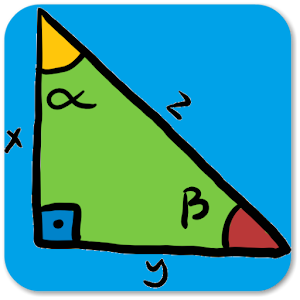# Math Formulas - Lumos Educational App Store31
Price -NA
\$A

#### DESCRIPTION:

This is a perfect app on Google Play that provides all basic formulas in mathematics. It's very convenient for all students in high school or university and engineers to look for any easy or complicated formulas. It includes: All essentials math formula and equations: Algebra Geometry Trigonometry Calculus: Limits Derivatives Integrals Basic Properties & Facts Arithmetic Operations Exponent Properties Properties of Radicals Properties of Inequalities Properties of Absolute Value Distance Formula Complex Numbers Logarithms and Log Properties Factoring and Solving Fa

#### OVERVIEW:

Math Formulas is a free educational mobile app By Svey Development.It helps students in grades HS practice the following standards HSF.LE.A.1,HSF.LE.A.2,HSF.LE.A.3.

This page not only allows students and teachers download Math Formulas but also find engaging Sample Questions, Videos, Pins, Worksheets, Books related to the following topics.

1. HSF.LE.A.1 : Distinguish between situations that can be modeled with linear functions and with exponential functions.

2. HSF.LE.A.2 : Construct linear and exponential functions, including arithmetic and geometric sequences, given a graph, a description of a relationship, or two input-output pairs.

3. HSF.LE.A.3 : Observe using graphs and tables that a quantity increasing exponentially eventually exceeds a quantity increasing linearly, quadratically, or (more generally) as a polynomial function..

HS

#### STANDARDS:

HSF.LE.A.1
HSF.LE.A.2
HSF.LE.A.3
HSF.LE.A.4
HSF.LE.B.5
HSF.TF.A.1
HSF.TF.A.2
HSF.TF.A.3
HSF.TF.A.4
HSF.TF.B.5
HSF.TF.B.6
HSF.TF.B.7
HSF.TF.C.8
HSF.TF.C.9

Developer: Svey Development

Software Version: 1.0.1

Category: Education

### RELATED APPSEdSearch WebSearch